# ∈-NFA of Regular Language L = {ab,ba}

• Difficulty Level : Basic
• Last Updated : 11 Nov, 2020

Prerequisite – Introduction of Finite Automata

Non-Deterministic Finite Automata and ∈-Non-Deterministic Finite Automata are almost the same except for their transition function and there are a few special rules for construction of ∈-NFA.

Attention reader! Don’t stop learning now.  Practice GATE exam well before the actual exam with the subject-wise and overall quizzes available in GATE Test Series Course.

Learn all GATE CS concepts with Free Live Classes on our youtube channel.

```∈-NFA is defined in 5 tuple representation {Q, q0, Σ, δ, F} where,
Q is the set of all states,
q0 is the initial state,
Σ is the set of input symbols,
δ is the transition function which is δ:Q × (Σ∪∈)->2Q and
F is the set of final states.
```

Simple rules for construction of ∈-NFA :

`∈-NFA for a+ :`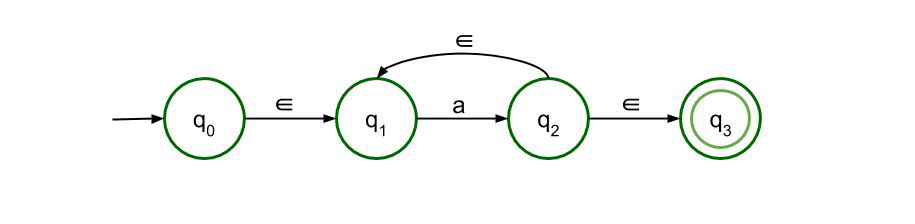This structure is for a+ which means there must be at least one a” in the expression. It is preceded by epsilon and also succeeded by one. There is epsilon feedback from state q2 to q1 so that there can be more than one a” in the expression.

`∈-NFA for a* :`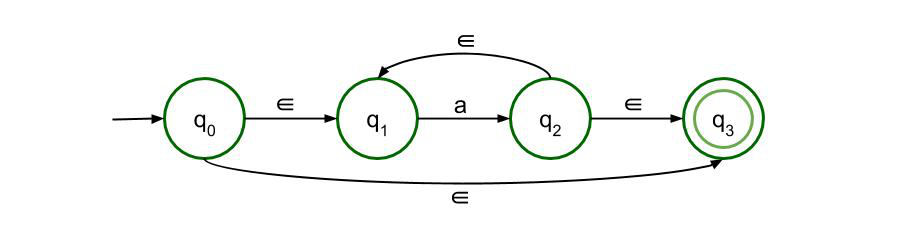This structure is for a* which means there can be any number of ‘a’ in the expression, even 0. The previous structure is just modified a bit so that even if there is no input symbol, i.e if the input symbol is null, then also the expression is valid.

`∈-NFA for a+b :`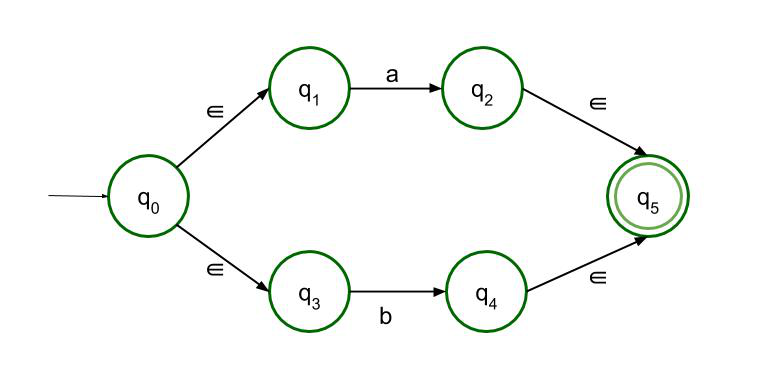This structure accepts either a or b as input. So there are two paths, both of which lead to the final state.

`∈-NFA for ab :`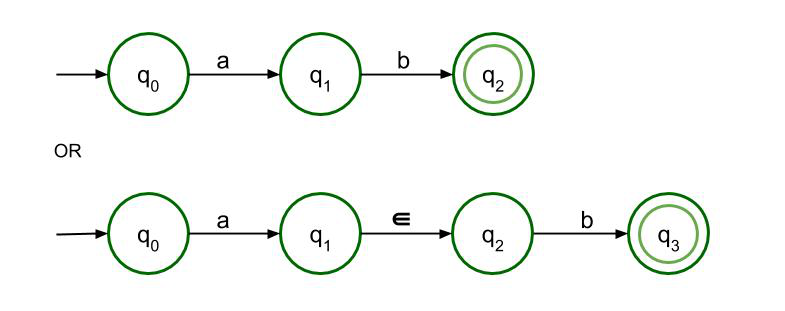For concatenation, a must be followed by b. Only then it can reach the final state. Both structures are allowed here but as it is ∈-NFA so the second structure is recommended.

∈-NFA for L = {ab, ba} :
Following the above-mentioned rules, ∈-NFA of Regular Language L ={ab, ba} is to be constructed.

The language consists of ab or ba, which can also be written as (ab + ba). Following the rule of constructing an addition or (a+b), we have to construct the main structure. However, here, ‘a’ in a+b is actually the expression ‘ab’ and ‘b’ in a+b is actually the expression ‘ba’. So, we just replace their structures in place of a and b respectively. So the final ∈-NFA will have two paths, one for ab and another for ba both of which lead to the final state.

Following the rule of constructing concatenation or ab, we can individually construct the structures for ab and ba. For concatenation, any one of the two above-mentioned structures, i.e, with epsilon or without epsilon, can be chosen but we choose the one with the epsilon because it is an ∈-NFA.

The Final ∈-NFA will be :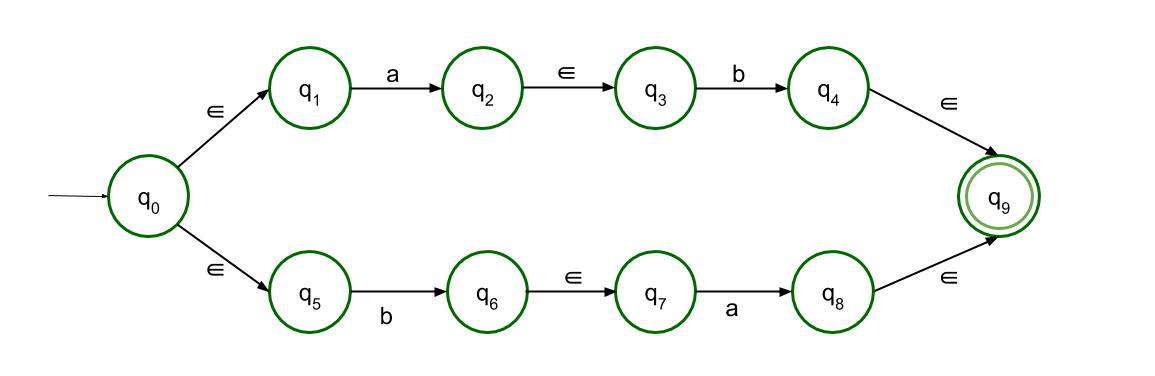My Personal Notes arrow_drop_up